图文详解Heap Sort堆排序算法及JavaScript的代码实现

1. 不得不说说二叉树（深度为 3 的满二叉树 full binary tree）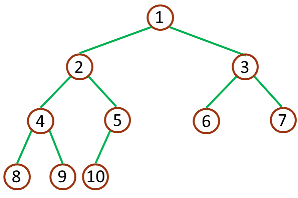（深度为 3 的完全二叉树 complete binary tree）
2. 什么是堆？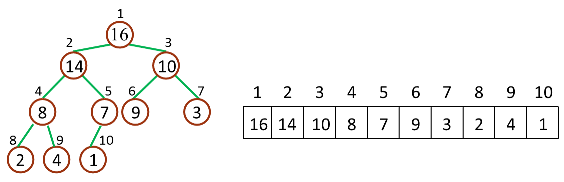（堆和数组的相互关系）

Parent(i) = floor(i/2)，i 的父节点下标
Left(i) = 2i，i 的左子节点下标
Right(i) = 2i + 1，i 的右子节点下标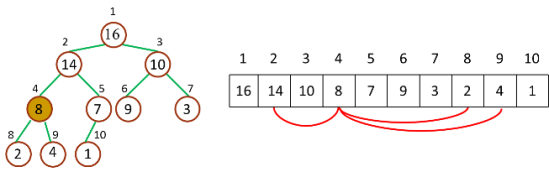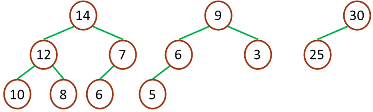（最大堆）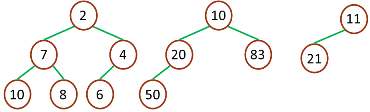（最小堆）
3. 堆排序原理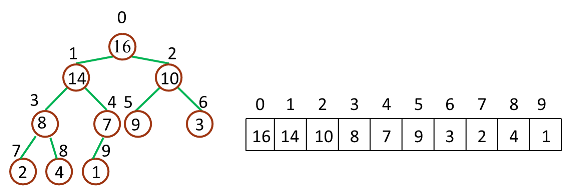（Zero-Based）

Parent(i) = floor((i-1)/2)，i 的父节点下标
Left(i) = 2i + 1，i 的左子节点下标
Right(i) = 2(i + 1)，i 的右子节点下标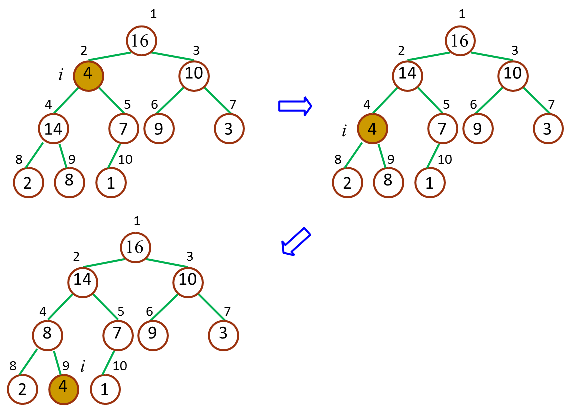（Max-Heapify）

```/**
* 从 index 开始检查并保持最大堆性质
*
* @array
*
* @index 检查的起始下标
*
* @heapSize 堆大小
*
**/
function maxHeapify(array, index, heapSize) {
var iMax = index,
iLeft = 2 * index + 1,
iRight = 2 * (index + 1);

if (iLeft < heapSize && array[index] < array[iLeft]) {
iMax = iLeft;
}

if (iRight < heapSize && array[iMax] < array[iRight]) {
iMax = iRight;
}

if (iMax != index) {
swap(array, iMax, index);
maxHeapify(array, iMax, heapSize); // 递归调整
}
}

function swap(array, i, j) {
var temp = array[i];
array[i] = array[j];
array[j] = temp;
}

```

```/**
* 从 index 开始检查并保持最大堆性质
*
* @array
*
* @index 检查的起始下标
*
* @heapSize 堆大小
*
**/
function maxHeapify(array, index, heapSize) {
var iMax, iLeft, iRight;
while (true) {
iMax = index;
iLeft = 2 * index + 1;
iRight = 2 * (index + 1);
if (iLeft < heapSize && array[index] < array[iLeft]) {
iMax = iLeft;
}

if (iRight < heapSize && array[iMax] < array[iRight]) {
iMax = iRight;
}

if (iMax != index) {
swap(array, iMax, index);
index = iMax;
} else {
break;
}
}
}

function swap(array, i, j) {
var temp = array[i];
array[i] = array[j];
array[j] = temp;
}

```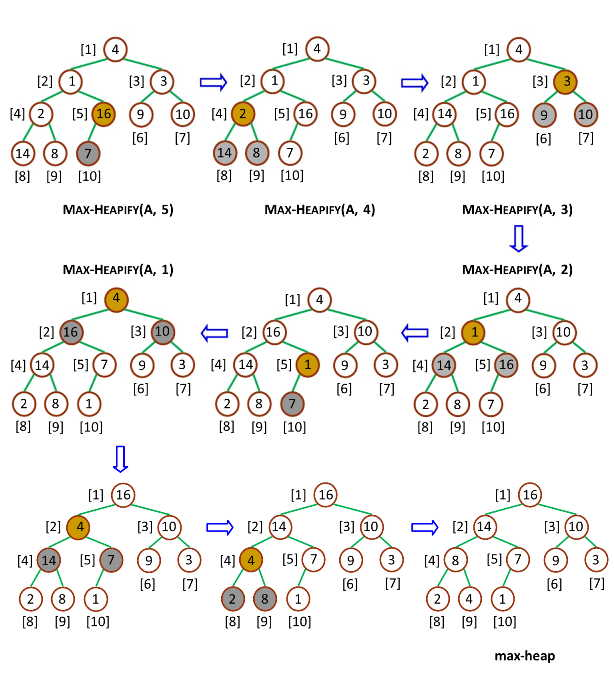```function buildMaxHeap(array, heapSize) {
var i,
iParent = Math.floor((heapSize - 1) / 2);

for (i = iParent; i >= 0; i--) {
maxHeapify(array, i, heapSize);
}
}
``````function heapSort(array, heapSize) {

buildMaxHeap(array, heapSize);

for (int i = heapSize - 1; i > 0; i--) {
swap(array, 0, i);
maxHeapify(array, 0, i);
}
}

```

4.JavaScript 语言实现

```function heapSort(array) {

function swap(array, i, j) {
var temp = array[i];
array[i] = array[j];
array[j] = temp;
}

function maxHeapify(array, index, heapSize) {
var iMax,
iLeft,
iRight;
while (true) {
iMax = index;
iLeft = 2 * index + 1;
iRight = 2 * (index + 1);

if (iLeft < heapSize && array[index] < array[iLeft]) {
iMax = iLeft;
}

if (iRight < heapSize && array[iMax] < array[iRight]) {
iMax = iRight;
}

if (iMax != index) {
swap(array, iMax, index);
index = iMax;
} else {
break;
}
}
}

function buildMaxHeap(array) {
var i,
iParent = Math.floor(array.length / 2) - 1;

for (i = iParent; i >= 0; i--) {
maxHeapify(array, i, array.length);
}
}

function sort(array) {
buildMaxHeap(array);

for (var i = array.length - 1; i > 0; i--) {
swap(array, 0, i);
maxHeapify(array, 0, i);
}
return array;
}

return sort(array);
}

```

5.堆排序算法的运用

（1）算法性能/复杂度

（2）算法稳定性

（3）算法适用场景

5个最顶级jQuery图表类库插件【jquery插件库】
GraphUpjQueryplugin-15美元Graphup是一中非常轻量级的灵活的jQuery(v1.4+)插件用来美化你的数据表。它能够使用颜色，柱状图及其气饱来有效的展现你的数据。

JavaScript中的继承比较奇葩，无法实现接口继承，只能依靠原型继承。原型链原型就是一个对象，通过构造函数创建出来的实例会有指针指向原型得到原

jQuery Mobile 和 Kendo UI 的比较
jQueryMobile和KendoUI都是流行的JavaScript框架，在开发中我们可以在它们的基础上添砖加瓦制作所有现代移动WEB应用。这两个框架都是基于使用率顶尖的JavaSc